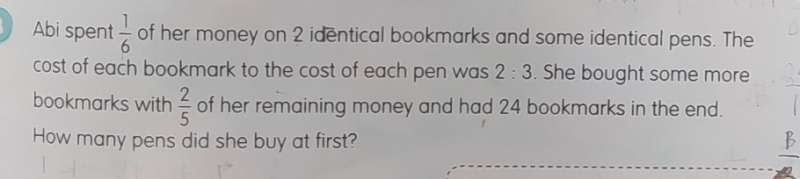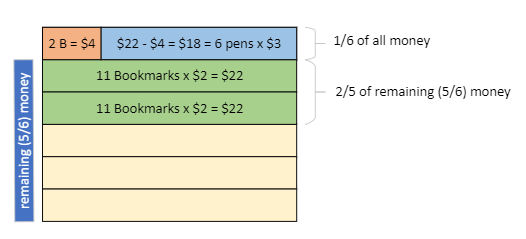# QuestionAbi spent of her money on 2 identical bookmarks and some identical pens. The cost of each bookmark to the cost of each pen was 2:3. She bought some more bookmarks with 2/5 of her remaining money and had 24 bookmarks in the end How many pens did she buy at first?

Source: Ang Mo Kio Primary

Here is how I would do it with block diagrams.Since the price ratio is 2:3, let’s assume a bookmark cost \$2 and a pen cost \$3.

• The remaining money is bottom 5 blocks.
• 2/5 of that is two green blocks.
• Two green blocks are used to pay for 24 – 2 = 22 bookmarks.
• Each green block is the cost of 11 bookmarks = 2 x 11 = \$22.
• Orange block is cost of 2 bookmarks = \$4.
• Blue block is 22 – 4 = \$18.
• So number of pens bought = \$18/\$3 = 6.

To be more general, we can say a bookmark costs 2u and a pen cost 3u. But really, a \$ is just another unit. So we can use \$ itself as the unit. Makes it easier to think about. You can replace the \$ above with “u” if you like. 🙂

0 Replies 1 Like

Book mark              Pen

Cost of each                   2u                        3u            Total spent = 1/6 of money

2/5 of remaining money(1-1/6=5/6) = 2/5   x   5/6    =  1/3

1/3 of money   buys (24-2)=   22 bookmarks which is  22 x 2u = \$44u

1/6 of money = \$44u  /  2  = \$22u

Cost of 2 bookmarks = 2 x 2u = 4u

So cost of pens = 22u-4u = 18u

Hence number of pens bought = 18u / 3u =6

0 Replies 1 Like

Assuming Abi had 6U of money at first.

After spending 1/6 of money or 1U, she was left with 5U

Then she used 2U for 24-2=22 bookmarks

So 1U can buy 11 bookmarks

Cost of a pen was 3/2 times of bookmarks or 1.5 bookmarks

Initially she spent 1U of money and can buy 11 bookmarks

11-2=9

9/1.5=6

So she bought 6 pens initially.

0 Replies 1 Like